JMSLTM Numerical Library 7.2.0
com.imsl.math

## Class MinUncon

• All Implemented Interfaces:
Serializable, Cloneable

```public class MinUncon
extends Object
implements Serializable, Cloneable```
Unconstrained minimization.

`MinUncon` uses two separate algorithms to compute the minimum depending on what the user supplies as the function `f`.

If `f` defines the function whose minimum is to be found `MinUncon` uses a safeguarded quadratic interpolation method to find a minimum point of a univariate function. Both the code and the underlying algorithm are based on the routine `ZXLSF` written by M.J.D. Powell at the University of Cambridge.

`MinUncon` finds the least value of a univariate function, f, where `f` implements `MinUnconFunction f`. Optional data include an initial estimate of the solution, and a positive number `bound`, specified by the `setBound` method. Let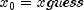where `xguess` is specified by the `setGuess` method and b = bound, then x is restricted to the interval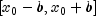. Usually, the algorithm begins the search by moving from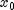to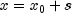, where s = step. `step` is set by the `setStep` method. If `setStep` is not called then `step` is set to 0.1. `step` may be positive or negative. The first two function evaluations indicate the direction to the minimum point, and the search strides out along this direction until a bracket on a minimum point is found or until x reaches one of the bounds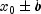. During this stage, the step length increases by a factor of between two and nine per function evaluation; the factor depends on the position of the minimum point that is predicted by quadratic interpolation of the three most recent function values.

When an interval containing a solution has been found, we will have three points,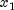,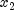, and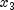, with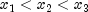and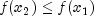and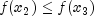. There are three main ingredients in the technique for choosing the new x from these three points. They are (i) the estimate of the minimum point that is given by quadratic interpolation of the three function values, (ii) a tolerance parameter, that depends on the closeness of f to a quadratic, and (iii) whetheris near the center of the range betweenandor is relatively close to an end of this range. In outline, the new value of x is as near as possible to the predicted minimum point, subject to being at leastfrom, and subject to being in the longer interval betweenandorandwhenis particularly close toor. There is some elaboration, however, when the distance between these points is close to the required accuracy; when the distance is close to the machine precision; or whenis relatively large.

The algorithm is intended to provide fast convergence when f has a positive and continuous second derivative at the minimum and to avoid gross inefficiencies in pathological cases, such as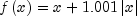The algorithm can makelarge automatically in the pathological cases. In this case, it is usual for a new value of x to be at the midpoint of the longer interval that is adjacent to the least calculated function value. The midpoint strategy is used frequently when changes to f are dominated by computer rounding errors, which will almost certainly happen if the user requests an accuracy that is less than the square root of the machine precision. In such cases, the routine claims to have achieved the required accuracy if it knows that there is a local minimum point within distance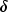of x, where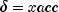, specified by the `setAccuracy` method even though the rounding errors in f may cause the existence of other local minimum points nearby. This difficulty is inevitable in minimization routines that use only function values, so high precision arithmetic is recommended.

If `f` implements `MinUnconDerivative` then `MinUncon` uses a descent method with either the secant method or cubic interpolation to find a minimum point of a univariate function. It starts with an initial guess and two endpoints. If any of the three points is a local minimum point and has least function value, the routine terminates with a solution. Otherwise, the point with least function value will be used as the starting point.

From the starting point, say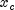, the function value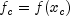, the derivative value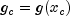, and a new point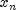defined by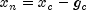are computed. The function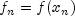, and the derivative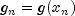are then evaluated. If either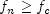or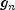has the opposite sign of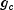, then there exists a minimum point betweenand; and an initial interval is obtained. Otherwise, sinceis kept as the point that has lowest function value, an interchange betweenandis performed. The secant method is then used to get a new point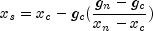Let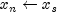and repeat this process until an interval containing a minimum is found or one of the convergence criteria is satisfied. The convergence criteria are as follows: Criterion 1: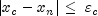Criterion 2: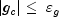where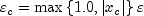,is a relative error tolerance and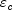is a gradient tolerance.

When convergence is not achieved, a cubic interpolation is performed to obtain a new point. Function and derivative are then evaluated at that point; and accordingly, a smaller interval that contains a minimum point is chosen. A safeguarded method is used to ensure that the interval reduces by at least a fraction of the previous interval. Another cubic interpolation is then performed, and this procedure is repeated until one of the stopping criteria is met.

Function values only Example, Function values and derivatives Example, Serialized Form
• ### Nested Class Summary

Nested Classes
Modifier and Type Class and Description
`static interface ` `MinUncon.Derivative`
Public interface for the user supplied function to the `MinUncon` object.
`static interface ` `MinUncon.Function`
Public interface for the user supplied function to the `MinUncon` object.
• ### Constructor Summary

Constructors
Constructor and Description
`MinUncon()`
Unconstrained minimum constructor for a smooth function of a single variable of type `double`.
• ### Method Summary

Methods
Modifier and Type Method and Description
`double` `computeMin(MinUncon.Function F)`
Return the minimum of a smooth function of a single variable of type `double` using function values only or using function values and derivatives.
`void` `setAccuracy(double xacc)`
Set the required absolute accuracy in the final value returned by member function `computeMin`.
`void` `setBound(double bound)`
Set the amount by which X may be changed from its initial value, xguess.
`void` `setDerivtol(double gtol)`
Set the derivative tolerance used by member function `computeMin` to decide if the current point is a local minimum.
`void` `setGuess(double xguess)`
Set the initial guess of the minimum point of the input function.
`void` `setStep(double step)`
Set the stepsize to use when changing x.
• ### Methods inherited from class java.lang.Object

`clone, equals, finalize, getClass, hashCode, notify, notifyAll, toString, wait, wait, wait`
• ### Constructor Detail

• #### MinUncon

`public MinUncon()`
Unconstrained minimum constructor for a smooth function of a single variable of type `double`.
• ### Method Detail

• #### computeMin

`public double computeMin(MinUncon.Function F)`
Return the minimum of a smooth function of a single variable of type `double` using function values only or using function values and derivatives.
Parameters:
`F` - defines the function whose minimum is to be found. If `F` implements `Derivative` then derivatives are used. Otherwise, an attempt to find the minimum is made using function values only.
Returns:
a `double` scalar value containing the minimum of the input function
• #### setAccuracy

`public void setAccuracy(double xacc)`
Set the required absolute accuracy in the final value returned by member function `computeMin`. If this member function is not called, the required accuracy is set to 1.0e-8.
Parameters:
`xacc` - a `double`scalar value specifying the required absolute accuracy in the final value returned by member function `computeMin`.
• #### setBound

`public void setBound(double bound)`
Set the amount by which X may be changed from its initial value, xguess. If this member function is not called, bound is set to 100.
Parameters:
`bound` - a `double` scalar value specifying the amount by which X may be changed from its initial value. In other words, X is restricted to the interval [`xguess-bound`, `xguess+bound`].
• #### setDerivtol

`public void setDerivtol(double gtol)`
Set the derivative tolerance used by member function `computeMin` to decide if the current point is a local minimum. This is the second stopping criterion. x is returned as a solution when G(x) is less than or equal to `gtol`. `gtol` should be nonnegative, otherwise zero will be used. If this member function is not called, the derivative tolerance is set to 1.0e-8.
Parameters:
`gtol` - a `double`scalar value specifying the derivative tolerance used by member function `computeMin`.
• #### setGuess

`public void setGuess(double xguess)`
Set the initial guess of the minimum point of the input function. If this member function is not called, an initial guess of 0.0 is used.
Parameters:
`xguess` - a `double` scalar value specifying the initial guess of the minimum point of the input function
• #### setStep

`public void setStep(double step)`
Set the stepsize to use when changing x. If this member function is not called, step is set to 0.1.
Parameters:
`step` - a `double` scalar value specifying the order of magnitude estimate of the required change in x when stepping towards the minimum
JMSLTM Numerical Library 7.2.0